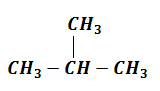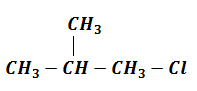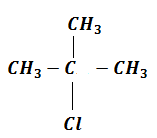$\left({\mathrm{CH}}_{3}{\right)}_{3}\mathrm{CH}$ + ${\mathrm{Cl}}_{2}\stackrel{\mathrm{hv}}{\to }$ A(Major), the compound 'A' is

(1 mole)

1. $\left({\mathrm{CH}}_{3}{\right)}_{2}\mathrm{CH}-{\mathrm{CH}}_{2}-\mathrm{Cl}$

2. $\left({\mathrm{CH}}_{3}{\right)}_{3}\mathrm{C}-\mathrm{Cl}$

3. Equimolar mixture of (1) and (2)

4. No reaction

Concept Videos :-

#12 | Differentiation: Alkanes, Alkenes & Alkynes
#13 | Differentiation: Assignment on Alkane to Alkyne
#18 | Addition Reaction: 1
#19 | Addition Reaction: 2
#6 | Chemical Properties of Alkane
#9 | Chemical Properties of Alkene:I
#10 | Reaction of Alkene:I
#11 | Reaction of Alkene:II
#14 | Preparation of Alkyne
#15 | Reaction of Alkyne:I

Concept Questions :-

Alkanes, Alkenes and Alkynes - Chemical Properties

(2) The reactivity order of abstraction of hydrogen is:${\mathrm{Cl}}_{2}\stackrel{\mathrm{hv}}{\to }$(1 mole)            (I)                              (II)

Posibility of (I) = No.of $1°\mathrm{H}$ $×$ Reactivity of $1°\mathrm{H}$ =9$×$1=9

Posibility of (II) = No.of $3°\mathrm{H}$ $×$ Reactivity of $3°\mathrm{H}$ =1$×$5=5

% of (I)=$\frac{9}{14}$ $×$100=64.3%

% of (II)=$\frac{5}{14}$ $×$100=35.7%

Difficulty Level:

• 13%
• 63%
• 20%
• 6%